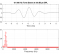## NLMS code (simple question)Started by 3 years ago7 replieslatest reply 3 years ago143 views

Hi all,

I'm implementing a NLMS filtering code and I'd like to confirm if I'm doing the right thing.

I'm evaluating the basic iterative equations (boldface for vectors, ^T for transposition, L for the order and n the iterations):

w(n) = w(n-1) + mu * x(n)^T * e(n)

e(n) = d(n) - x(n)*w(n-1)

x(n) = [x(n) x(n-1) ... x(n-L+1)]^T

by means of a for-loop that goes from n=L until n=length(d), after initializing all variables with zeros.

My supposition is that the first computation of x(n) occurs when n=L. Is it correct?

Also, I was wondering if I should initialize the error vector with:

e(1:L-1) = d(1:L-1)   -> the change I probably have to make

e(1:L-1) = 0             -> the way my code currently works

Any help is appreciated.

Best regards,

Artur

[ - ]I typically do this the other way around, i.e.

x(n) = [x(n) x(n-1) ... x(n-L+1)]^T

e(n) = d(n) - x(n)*w(n)

w(n+1) = w(n) + mu * x(n)^T * e(n)

Then there is no need to initialise the error vector.

You can wait for n to equal L but it shouldn't be necessary.

[ - ]Considering the sequence of equations that you proposed, what is the range for n?

[ - ]Are you asking if n starts at zero? If so then yes. I would typically define negative x(i) = 0 (i.e. initialise x(0) to be zero)

[ - ]OK, perfect. Thanks!
[ - ]NLMS or LMS?
[ - ]